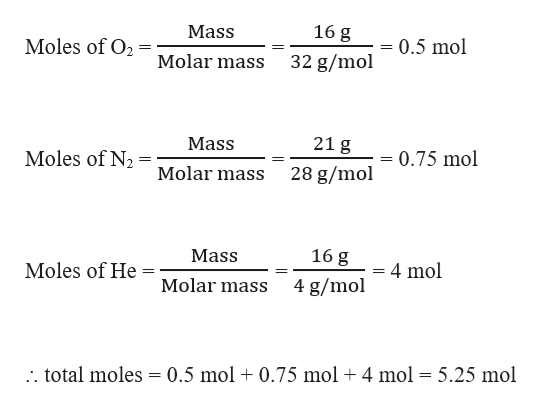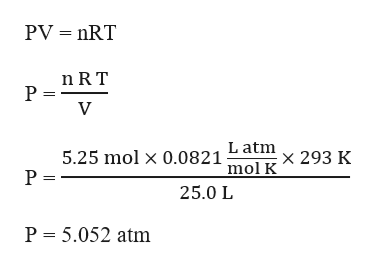# A gaseous mixture composed of 16.0 g of O_{2}2​, 21.0 g of N_{2}2​, and 16.0 g of He at 20.0°C is confined in a 25.0 L container. What is the partial pressure of He in this mixture?

Question
878 views

A gaseous mixture composed of 16.0 g of O_{2}2​, 21.0 g of N_{2}2​, and 16.0 g of He at 20.0°C is confined in a 25.0 L container. What is the partial pressure of He in this mixture?

check_circle

Step 1

Given,

Mass of O2 = 16 g

Mass of N2 = 21 g

Mass of He = 16 g

Temperature, T = 20.0°C = (20+273) K = 293 K

Volume, V = 25.0 L

Total moles of O2 , N2 and He can be calculated as:help_outlineImage Transcriptionclose16 g Mass Moles of O2 0.5 mol Molar mass 32 g/mol 21 g Mass Moles of N2 0.75 mol Molar mass 28 g/mol 16 g Mass Moles of He 4 mol Molar mass 4 g/mol . total moles = 0.5 mol 0.75 mol 4 mol = 5.25 mol fullscreen
Step 2

The total pressure can be calculated using the ideal gas equation as:help_outlineImage TranscriptionclosePV nRT n RT P = V 5.25 mol x 0.0821Latm P = x 293 K mol K 25.0 L P 5.052 atm fullscreen
Step 3

The mole fraction He can ...

### Want to see the full answer?

See Solution

#### Want to see this answer and more?

Solutions are written by subject experts who are available 24/7. Questions are typically answered within 1 hour.*

See Solution
*Response times may vary by subject and question.
Tagged in

### Ideal gases